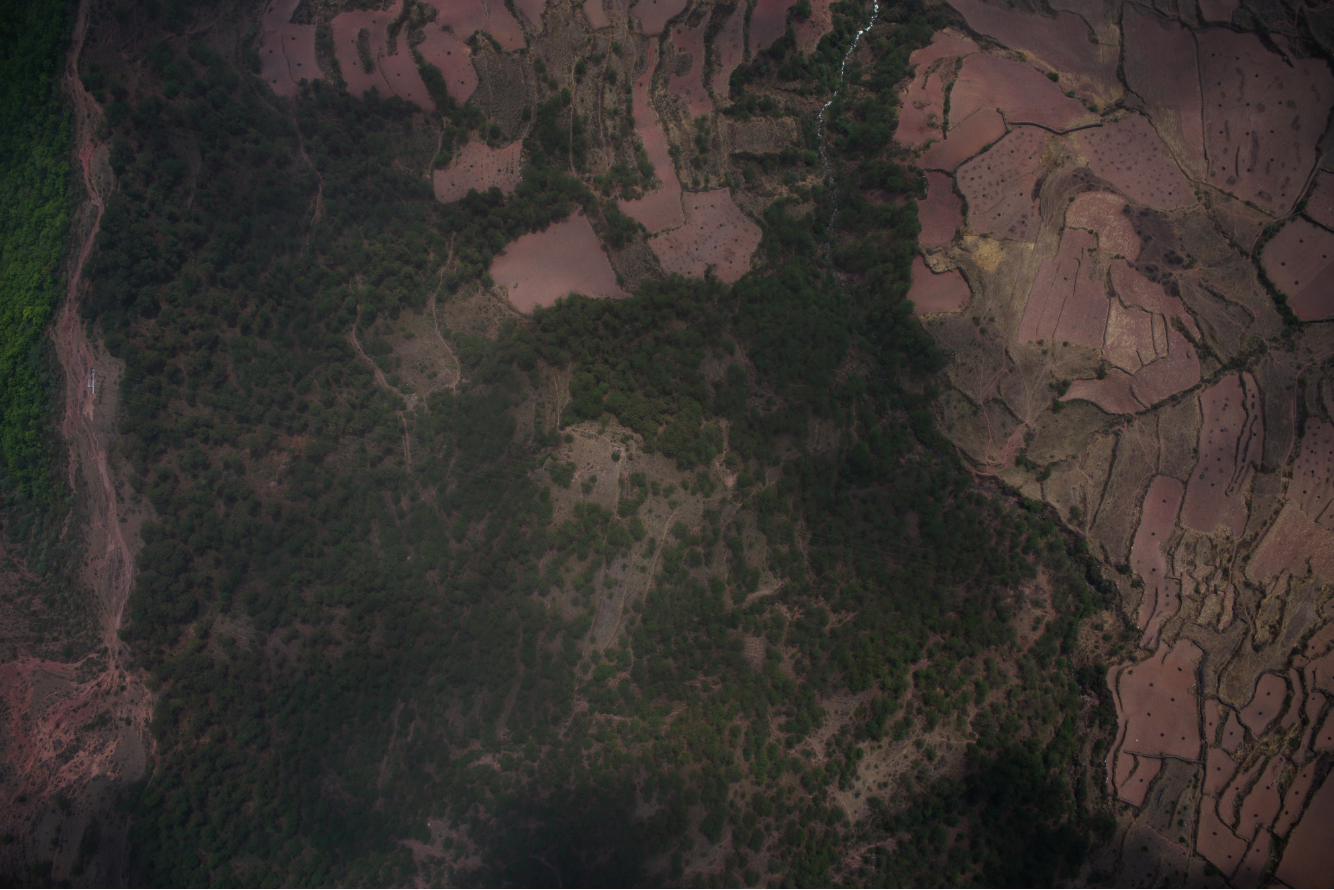2019-08-07 10:35:08 baidu_34971492 阅读数 147
• ###### MATLAB图像处理

全面系统的学习MATLAB在图像处理中的应用

19886 人正在学习 去看看 魏伟

# 基于FPGA的灰度图像处理之幂律（伽马）变化

## 1 背景知识

（1）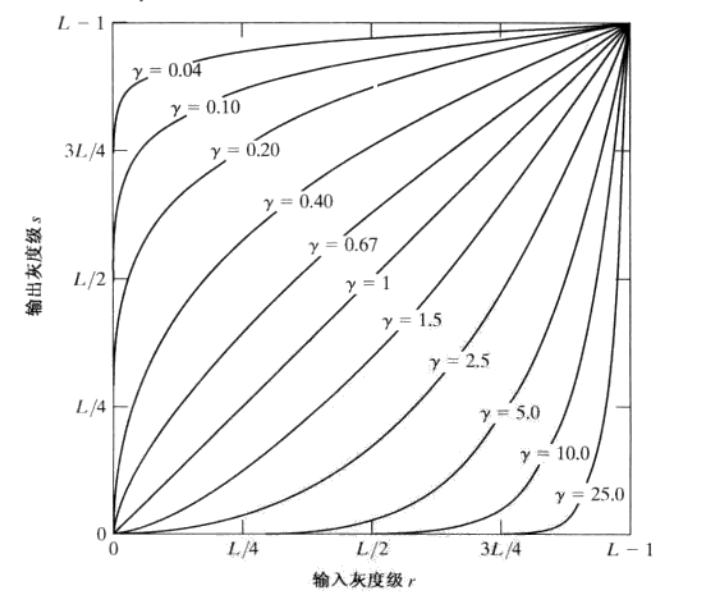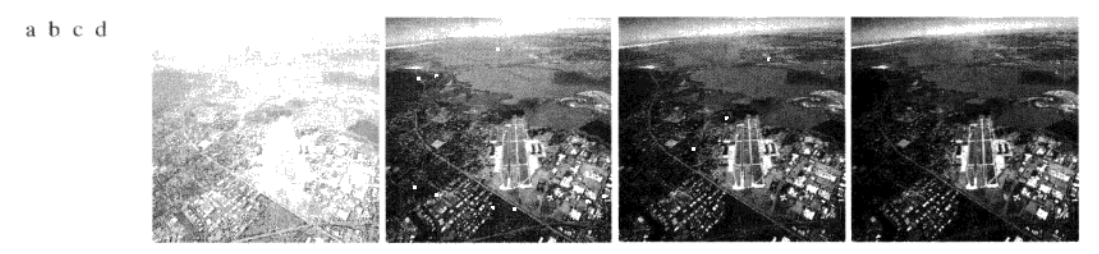## 2 FPGA实现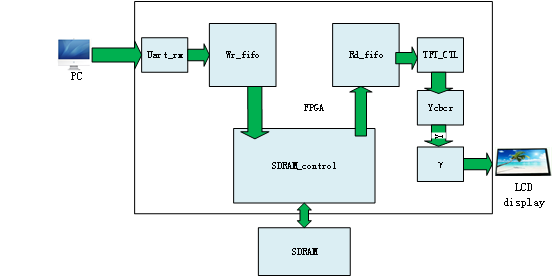ROM表的制作：
Matlab源码：

clear all
close all
clc
depth = 256;
width =8;
r = [0:1:255];
x = r;        %恒等变换
y =16*sqrt(r);%开根
%z = round(y);
m = (1/256)*r.^2;     %r平方
z = round(m);
fid = fopen('E:\matlab_project\log\square.mif','w');%路径
fprintf(fid,'depth= %d; \n',depth);
fprintf(fid,'width= %d; \n',width);
fprintf(fid,'Content Begin \n');
for(k=1:depth)
fprintf(fid,'%d: %d; \n',k-1,z(k));
end
fprintf(fid,'end;');
hold on
plot(x);
plot(y);
plot(m);
hold off


### FPGA源码：

//------------------------------------------
// power law
//------------------------------------------
wire [7:0]	 sqrt_data; //root
wire [7:0]	 square_data;//square
rom_sqrt rom_sqrt_inst(
.clken(TFT_de),
.clock(TFT_clk),
.q(sqrt_data)
);

rom_square rom_square_inst(
.clken(TFT_de),
.clock(TFT_clk),
.q(square_data)
);

//assign TFT_rgb = {sqrt_data[7:3],sqrt_data[7:2],sqrt_data[7:3]};     //Y
assign TFT_rgb = {square_data[7:3],square_data[7:2],square_data[7:3]};     //Y
//assign TFT_rgb = {o_y_8b[7:3],o_y_8b[7:2],o_y_8b[7:3]};     //Y


IP设置：

### 实验结果：2019-02-03 14:54:15 qq_42505705 阅读数 1378
• ###### MATLAB图像处理

全面系统的学习MATLAB在图像处理中的应用

19886 人正在学习 去看看 魏伟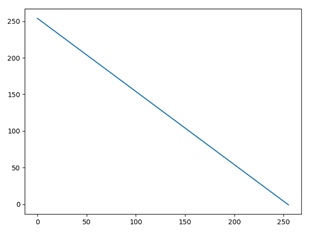import cv2
import numpy as np

reverse_img = 255 - img

cv2.imshow('srcimg',img)
cv2.imshow('reverse_img',reverse_img)
cv2.waitKey(0)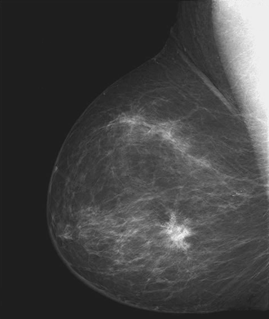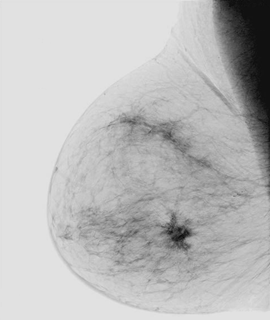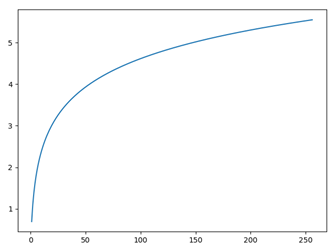import cv2
import math
import numpy as np

def logTransform(c,img):

#3通道RGB
'''h,w,d = img.shape,img.shape,img.shape
new_img = np.zeros((h,w,d))
for i in range(h):
for j in range(w):
for k in range(d):
new_img[i,j,k] = c*(math.log(1.0+img[i,j,k]))'''

#灰度图专属
h,w = img.shape, img.shape
new_img = np.zeros((h, w))
for i in range(h):
for j in range(w):
new_img[i, j] = c * (math.log(1.0 + img[i, j]))

new_img = cv2.normalize(new_img,new_img,0,255,cv2.NORM_MINMAX)

return new_img

#替换为你的图片路径

log_img = logTransform(1.0,img)
cv2.imshow('log_img',log_img)
cv2.imwrite(r'C:\Users\xxx\Desktop\Fourier spectrum2.jpg',log_img)
cv2.waitKey(0)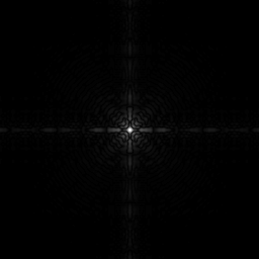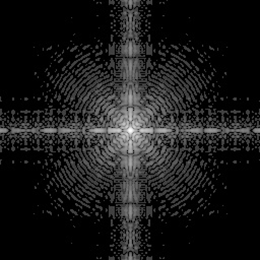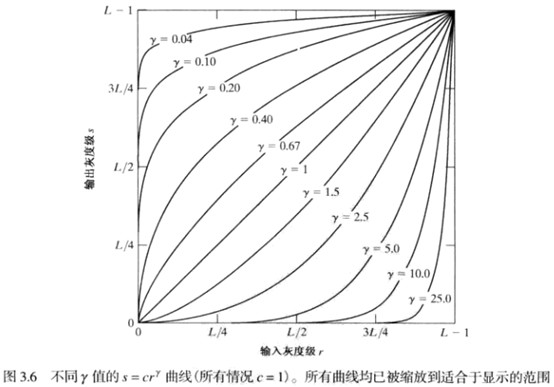import math
import numpy as np
import cv2

def gammaTranform(c,gamma,image):
h,w,d = image.shape,image.shape,image.shape
new_img = np.zeros((h,w,d),dtype=np.float32)
for i in range(h):
for j in range(w):
new_img[i,j,0] = c*math.pow(image[i, j, 0], gamma)
new_img[i,j,1] = c*math.pow(image[i, j, 1], gamma)
new_img[i,j,2] = c*math.pow(image[i, j, 2], gamma)
cv2.normalize(new_img,new_img,0,255,cv2.NORM_MINMAX)
new_img = cv2.convertScaleAbs(new_img)

return new_img

new_img = gammaTranform(1,2.5,img)

cv2.imshow('x',new_img)
cv2.imwrite(r'C:\Users\xxx\Desktop\gray_2.5.jpg',new_img)
cv2.waitKey(0)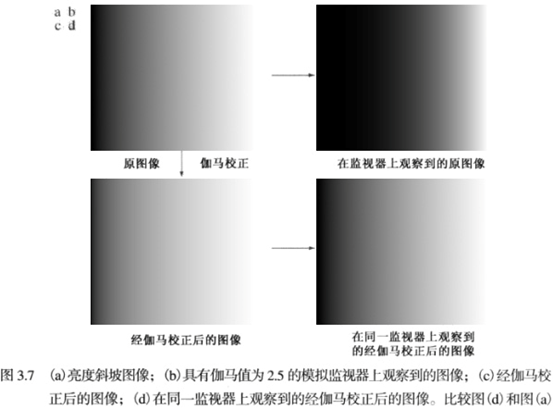γ<1 增强亮度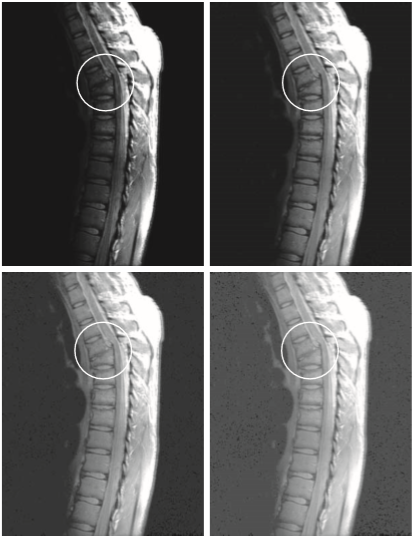γ>1 增强暗度2010-05-23 14:49:00 yangfenghero 阅读数 3459
• ###### MATLAB图像处理

全面系统的学习MATLAB在图像处理中的应用

19886 人正在学习 去看看 魏伟

幂次变换的基本表达式为：y=cxr+b

其中cr均为正数。与对数变换相同，幂次变换将部分灰度区域映射到更宽的区域中。当r=1时，幂次变换转变为线性变换。

(1)  当r<0时，变换函数曲线在正比函数上方。此时扩展低灰度级，压缩高灰度级，使图像变亮。这一点与对数变换十分相似。

(2)  当r>0时，变换函数曲线在正比函数下方。此时扩展高灰度级，压缩低灰度级，使图像变暗。

代码如下：

2019-11-14 20:11:58 qq_41398808 阅读数 124
• ###### MATLAB图像处理

全面系统的学习MATLAB在图像处理中的应用

19886 人正在学习 去看看 魏伟

>1的值所生成的曲线和<1所生成的曲线的效果完全相反，当c==1时简化为了恒等变换。导入要使用的第三方库：

from PIL import Image
import numpy as np
import matplotlib.pyplot as plt

读取图片数据，并可视化：

img = Image.open('小亮点.jpg')
plt.axis('off')
plt.imshow(img)
plt.show()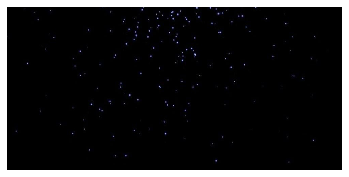图像数据转换为numpy数组：

img_data = np.array(img)

定义幂律变换函数：

def power_law_rollovers(img, func, c, b):
img_data = np.array(img)
a = np.shape(img_data)
new_img = []
for i in range(a):
new_row = []
for j in range(a):
data = list(img_data[i][j])
new_data = func(data, c, b)
new_row.append(np.array(new_data))
new_img.append(np.array(new_row))
return new_img
def power_law(data, c, b):
new_data = []
for k in data:
a = int(c*(k**b))
new_data.append(a)
return new_data

结果：

new_img1 = power_law_rollovers(img, power_law, 1, 0.8)
new_img2 = power_law_rollovers(img, power_law, 1, 0.6)
new_img3 = power_law_rollovers(img, power_law, 1, 0.4)
new_img4 = power_law_rollovers(img, power_law, 1, 0.3)

new_img1 = np.array(new_img1)
new_img2 = np.array(new_img2)
new_img3 = np.array(new_img3)
new_img4 = np.array(new_img4)

new_img1 = Image.fromarray(new_img1.astype('uint8')).convert('RGB')
new_img2 = Image.fromarray(new_img2.astype('uint8')).convert('RGB')
new_img3 = Image.fromarray(new_img3.astype('uint8')).convert('RGB')
new_img4 = Image.fromarray(new_img4.astype('uint8')).convert('RGB')

plt.figure(figsize=(25,60))
plt.subplot(221)
plt.axis('off')
gray1 = new_img1.convert('L')
plt.imshow(gray1, cmap='gray')
plt.subplot(222)
plt.axis('off')
gray2 = new_img2.convert('L')
plt.imshow(gray2, cmap='gray')
plt.subplot(223)
plt.axis('off')
gray3 = new_img3.convert('L')
plt.imshow(gray3, cmap='gray')
plt.subplot(224)
plt.axis('off')
gray4 = new_img4.convert('L')
plt.imshow(gray4, cmap='gray')
plt.show()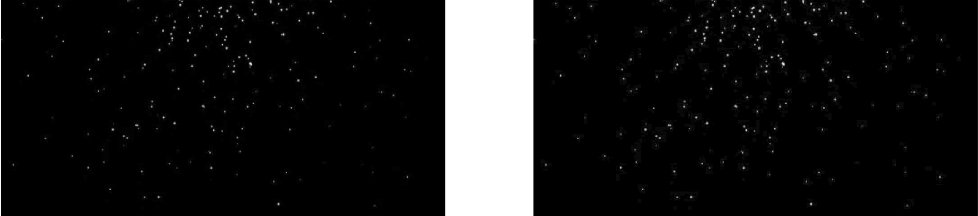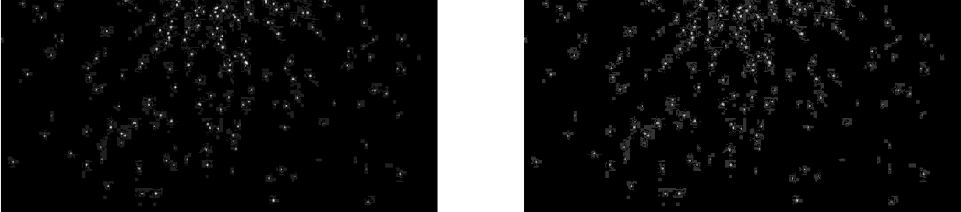2019-04-23 21:57:02 jameschen9051 阅读数 293
• ###### MATLAB图像处理

全面系统的学习MATLAB在图像处理中的应用

19886 人正在学习 去看看 魏伟

幂律(伽马)变换公式：，γ取值范围（0，+∞）

对图像做幂律变换处理，当γ取值（0,1）可以将图像较暗的灰度值映射到更大的灰度值，同时较亮部分变化较小；γ取值（1，+∞）可以将较亮部分映射到更低的亮度，而更暗的部分变化较小。

处理彩色图像，只需要将RGB取出，分别进行幂律变换即可。公式中，c可以取值255，r = a/255，a为像素上RGB分量之一，那么整个公式的取值范围为（0,255），不考虑计算过程的四舍五入的话，幂律变换的操作是可逆的。

public void gammaTranverse(BufferedImage image, double param) {
for (int i = 0; i < image.getWidth(); i++) {
for (int j = 0; j < image.getHeight(); j++) {

// 将rgb转换为各个值
int rgb = image.getRGB(i, j);
double R = (rgb >> 16) & 0xff;
double G = (rgb >> 8) & 0xff;
double B = rgb & 0xff;

// 进行伽马变换
R = 255 * Math.pow(R / 255, param);
G = 255 * Math.pow(G / 255, param);
B = 255 * Math.pow(B / 255, param);
// 将值转换为rgb
rgb = ((clamp(255) & 0xff) << 24) | ((clamp((int) R) & 0xff) << 16) | ((clamp((int) G) & 0xff) << 8)
| ((clamp((int) B) & 0xff));
image.setRGB(i, j, rgb);
}
}
}

1、原图。这是一张光线暗到几乎很难直接看到地面物体的航拍影像。通过幂律变换进行处理，增强较暗影像。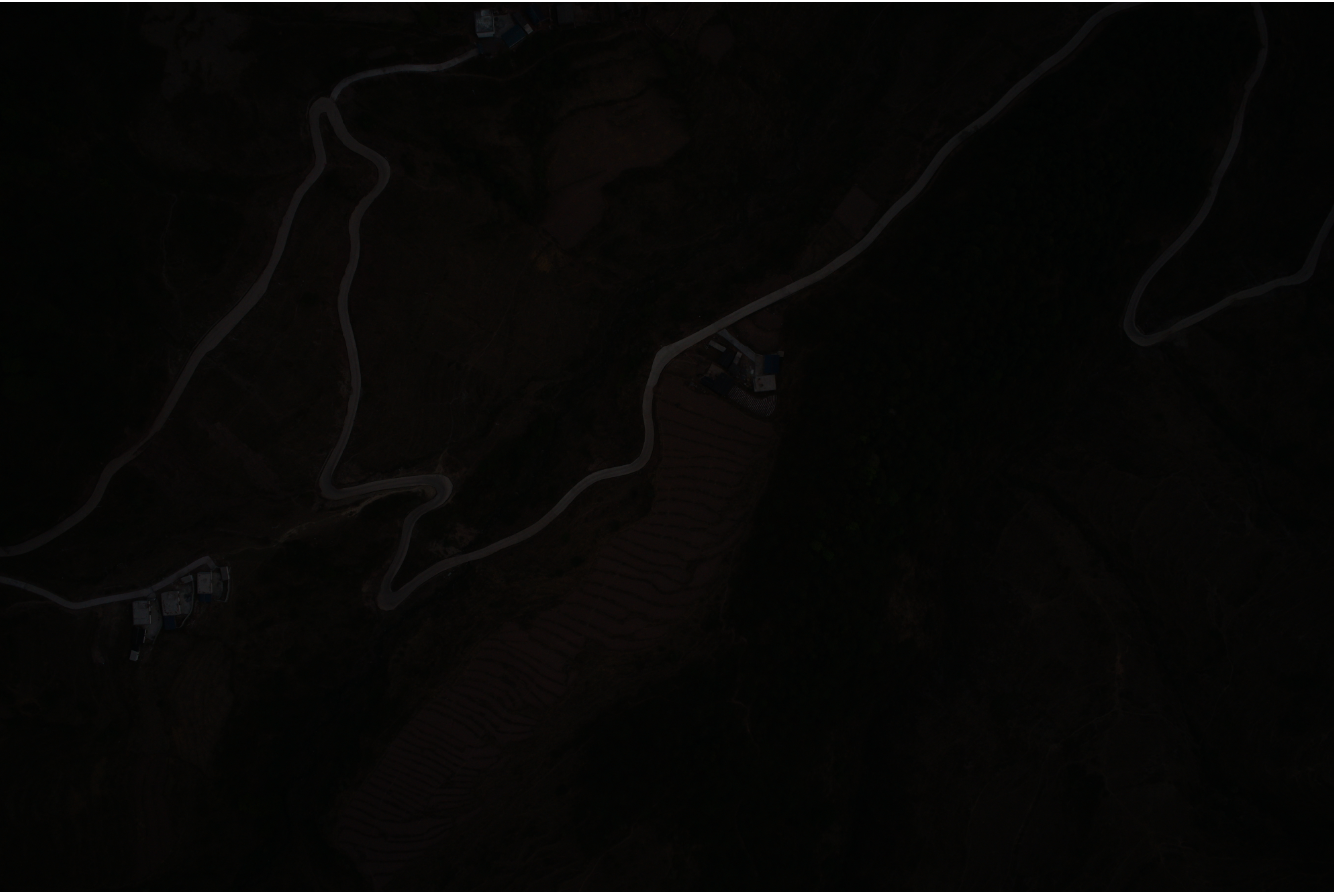2、γ取值为0.4，通过幂律变换处理后效果，可以看到影像增强效果非常明显，影像大部分区域由几乎不可见变得可见。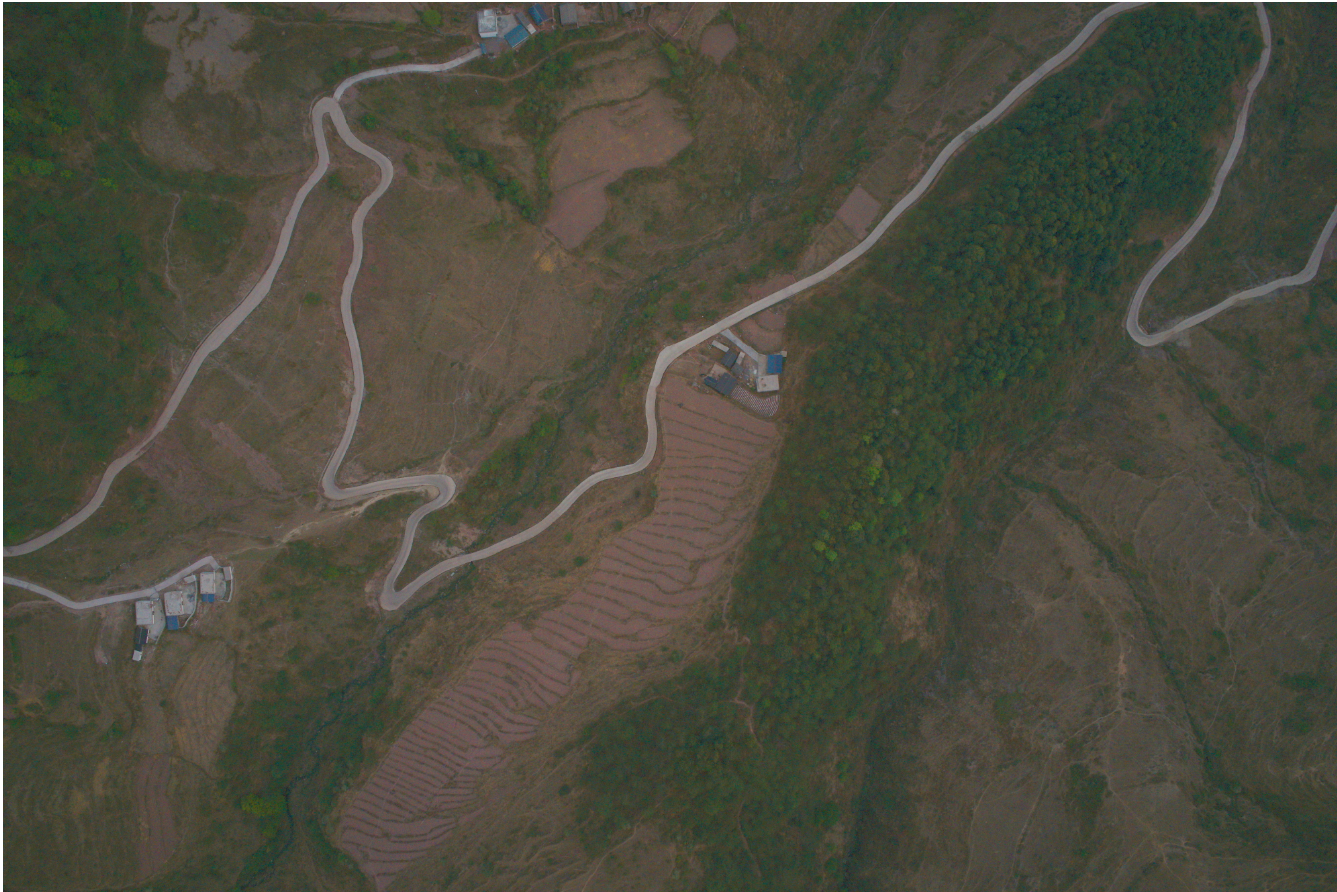3、这是一幅光线比较强，且受到云雾干扰的影像。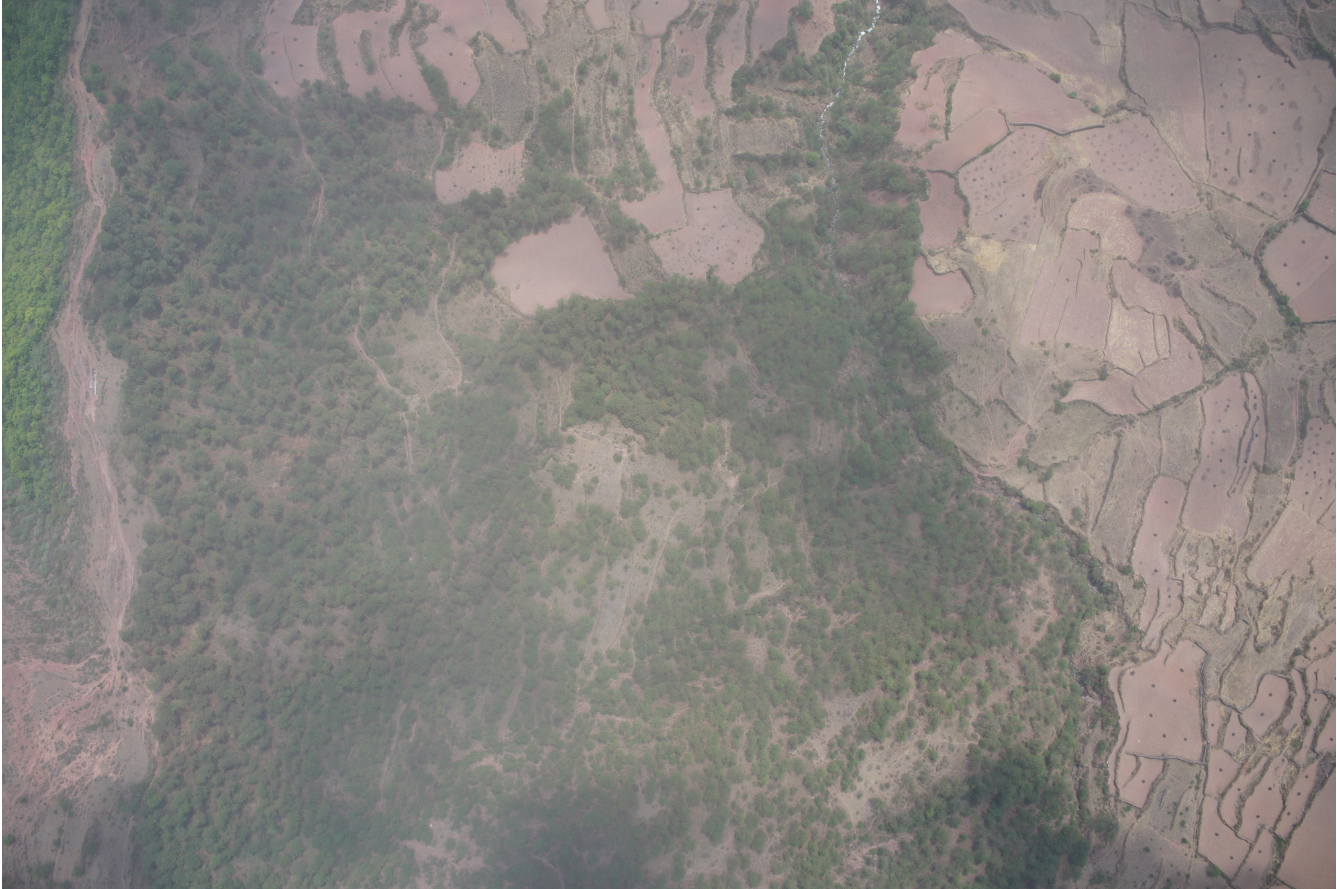4、γ取值为2处理后效果，影像整体变暗，但对比度更明显，受光线和云雾干扰造成的泛白现象减弱，整体视觉效果有所提升。